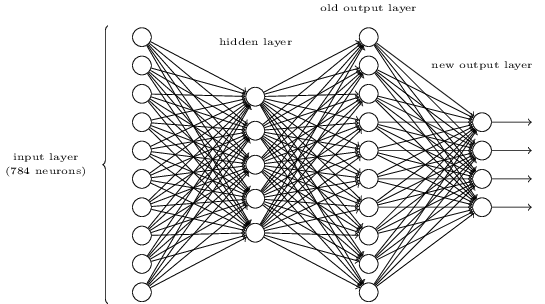Back to overviewNeural Net Problems - Exercise 2
March 22, 2020

Here are my solutions to exercise 2.

## The Architecture of Neural Networks

### Question

There is a way of determining the bitwise representation of a digit by adding an extra layer to the three-layer network above. The extra layer converts the output from the previous layer into a binary representation, as illustrated in the figure below. Find a set of weights and biases for the new output layer. Assume that the first layers of neurons are such that the correct output in the third layer (i.e., the old output layer) has activation at least , and incorrect outputs have activation less than .

### Solution

This is converting a base-ten value to base-two value.

I first listed out all the possible numbers.

#### Node One

I noticed that the first thing I could do it solve for the first binary digit. The first binary digit tells us whether or not the number is odd or even. I found that the bias is unnecessary since the dot product will produce either or . The weights for the first node are as follows:

I am going to denote the weight due to number in the old output layer, ,

So if the value is odd the and if even .

#### Node Two

For node two it should only be activated if the numbers are

#### Node Three

For node three it should only be activated if the numbers are

#### Node Four

For node four it should only be activated if the numbers are

Again, there are no biases for any of the nodes.